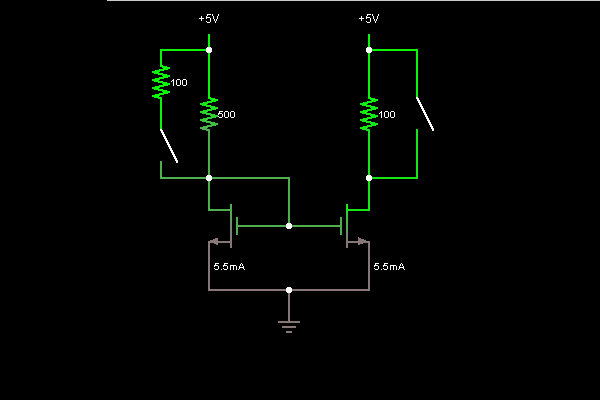# Circuit Simulator - Current Mirror (with MOSFETs)

## Where can I get Current Mirror (with MOSFETs) Circuit Diagram with Explanation?

IndiaBIX provides you lots of fully solved Current Mirror (with MOSFETs) circuit diagram with detailed explanation and working principles.

## How to design a Current Mirror (with MOSFETs) (electronic circuit)?

You can easily design the Current Mirror (with MOSFETs) circuit by practicing the exercises given below. Here you can design and simulate your own electronic circuits with this Online Circuit Designer and Simulator.### Circuit Description:

This is a current mirror, a device that uses the current in one half of the circuit to control the current flow in the other half. The current is the same in both halves. The switch on the left changes the current flow in the left half, which is mirrored in the right half. The switch on the right causes the resistor to be bypassed, but the current mirror ensures that the flow of current does not change.

If the left MOSFET is in saturation mode, its current is determined only by the gate-source voltage. The left MOSFET has its gate and drain tied together, so the drain voltage determins the current. The right MOSFET has the same gate-source voltage, so the current must be the same, as long as it is in saturation mode. -- Credits: Mr. Paul Falstad.

 Shaun said: (Aug 24, 2011) Awesome, thanks!